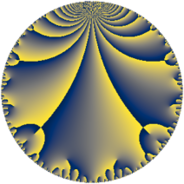# Properties

 Label 50.6.aLevel $50$ Weight $6$ Character orbit 50.a Rep. character $\chi_{50}(1,\cdot)$ Character field $\Q$ Dimension $7$ Newform subspaces $7$ Sturm bound $45$ Trace bound $3$

# Related objects

## Defining parameters

 Level: $$N$$ $$=$$ $$50 = 2 \cdot 5^{2}$$ Weight: $$k$$ $$=$$ $$6$$ Character orbit: $$[\chi]$$ $$=$$ 50.a (trivial) Character field: $$\Q$$ Newform subspaces: $$7$$ Sturm bound: $$45$$ Trace bound: $$3$$ Distinguishing $$T_p$$: $$3$$

## Dimensions

The following table gives the dimensions of various subspaces of $$M_{6}(\Gamma_0(50))$$.

Total New Old
Modular forms 43 7 36
Cusp forms 31 7 24
Eisenstein series 12 0 12

The following table gives the dimensions of the cuspidal new subspaces with specified eigenvalues for the Atkin-Lehner operators and the Fricke involution.

$$2$$$$5$$FrickeDim.
$$+$$$$+$$$$+$$$$1$$
$$+$$$$-$$$$-$$$$2$$
$$-$$$$+$$$$-$$$$3$$
$$-$$$$-$$$$+$$$$1$$
Plus space$$+$$$$2$$
Minus space$$-$$$$5$$

## Trace form

 $$7q + 4q^{2} - 4q^{3} + 112q^{4} + 8q^{6} + 312q^{7} + 64q^{8} + 221q^{9} + O(q^{10})$$ $$7q + 4q^{2} - 4q^{3} + 112q^{4} + 8q^{6} + 312q^{7} + 64q^{8} + 221q^{9} + 814q^{11} - 64q^{12} - 114q^{13} + 176q^{14} + 1792q^{16} - 918q^{17} + 3892q^{18} + 5590q^{19} + 3284q^{21} - 3312q^{22} - 3144q^{23} + 128q^{24} - 4152q^{26} + 5480q^{27} + 4992q^{28} - 7890q^{29} - 9036q^{31} + 1024q^{32} - 24288q^{33} - 13744q^{34} + 3536q^{36} + 16902q^{37} + 17360q^{38} - 15168q^{39} - 32636q^{41} - 11392q^{42} - 13164q^{43} + 13024q^{44} - 4912q^{46} - 44448q^{47} - 1024q^{48} + 16599q^{49} + 47354q^{51} - 1824q^{52} + 44406q^{53} + 680q^{54} + 2816q^{56} + 33040q^{57} - 37320q^{58} - 10180q^{59} + 22514q^{61} + 20768q^{62} + 42376q^{63} + 28672q^{64} - 2984q^{66} - 10788q^{67} - 14688q^{68} + 70892q^{69} + 64464q^{71} + 62272q^{72} + 9666q^{73} - 46984q^{74} + 89440q^{76} + 28464q^{77} - 112576q^{78} + 140860q^{79} - 91633q^{81} + 12648q^{82} + 220476q^{83} + 52544q^{84} - 153232q^{86} - 233880q^{87} - 52992q^{88} - 245720q^{89} - 1696q^{91} - 50304q^{92} - 117968q^{93} + 66496q^{94} + 2048q^{96} + 224682q^{97} - 2652q^{98} - 504008q^{99} + O(q^{100})$$

## Decomposition of $$S_{6}^{\mathrm{new}}(\Gamma_0(50))$$ into newform subspaces

Label Dim. $$A$$ Field CM Traces A-L signs $q$-expansion
$$a_2$$ $$a_3$$ $$a_5$$ $$a_7$$ 2 5
50.6.a.a $$1$$ $$8.019$$ $$\Q$$ None $$-4$$ $$-11$$ $$0$$ $$-142$$ $$+$$ $$-$$ $$q-4q^{2}-11q^{3}+2^{4}q^{4}+44q^{6}-142q^{7}+\cdots$$
50.6.a.b $$1$$ $$8.019$$ $$\Q$$ None $$-4$$ $$-6$$ $$0$$ $$118$$ $$+$$ $$+$$ $$q-4q^{2}-6q^{3}+2^{4}q^{4}+24q^{6}+118q^{7}+\cdots$$
50.6.a.c $$1$$ $$8.019$$ $$\Q$$ None $$-4$$ $$14$$ $$0$$ $$158$$ $$+$$ $$-$$ $$q-4q^{2}+14q^{3}+2^{4}q^{4}-56q^{6}+158q^{7}+\cdots$$
50.6.a.d $$1$$ $$8.019$$ $$\Q$$ None $$4$$ $$-24$$ $$0$$ $$172$$ $$-$$ $$+$$ $$q+4q^{2}-24q^{3}+2^{4}q^{4}-96q^{6}+172q^{7}+\cdots$$
50.6.a.e $$1$$ $$8.019$$ $$\Q$$ None $$4$$ $$-14$$ $$0$$ $$-158$$ $$-$$ $$-$$ $$q+4q^{2}-14q^{3}+2^{4}q^{4}-56q^{6}-158q^{7}+\cdots$$
50.6.a.f $$1$$ $$8.019$$ $$\Q$$ None $$4$$ $$11$$ $$0$$ $$142$$ $$-$$ $$+$$ $$q+4q^{2}+11q^{3}+2^{4}q^{4}+44q^{6}+142q^{7}+\cdots$$
50.6.a.g $$1$$ $$8.019$$ $$\Q$$ None $$4$$ $$26$$ $$0$$ $$22$$ $$-$$ $$+$$ $$q+4q^{2}+26q^{3}+2^{4}q^{4}+104q^{6}+\cdots$$

## Decomposition of $$S_{6}^{\mathrm{old}}(\Gamma_0(50))$$ into lower level spaces

$$S_{6}^{\mathrm{old}}(\Gamma_0(50)) \cong$$ $$S_{6}^{\mathrm{new}}(\Gamma_0(5))$$$$^{\oplus 4}$$$$\oplus$$$$S_{6}^{\mathrm{new}}(\Gamma_0(10))$$$$^{\oplus 2}$$$$\oplus$$$$S_{6}^{\mathrm{new}}(\Gamma_0(25))$$$$^{\oplus 2}$$